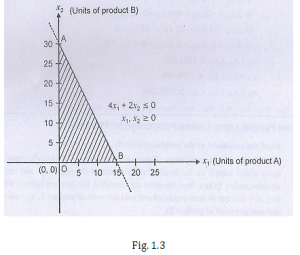2 products are manufactured in a company. It is through one department that the goods must be processed. The time required to produceper unit of product A is 4 hours whereas the production time for per unit of product B is 2 hours. 60 hours is calculated as total production time in the coming week.One of the production planning constraints is the total number of hours of two commodities which cannot surpass then time limit. There cannot be a negative variable as each of them are a representation of production quantity.Find the product combination of A and B that can be produced in entirety?

Solution

Total number of produced units in product A be X1

Total number of produced units in product B be X2

The constraint is represented by,

4 X1+ 2 X2 ≤60

This problem also suggests that,

X1 ≥0 and X2  ≥0

In the constraint equation 4 X1+ 2 X2 ≤60, different values can be put in one variable. The graphical representation can be showed by,

Point A:

X2= 0, X1= 15 which is placed at X-axis

Point B:

X1= 0, X2= 30 which is placed at Y-axis

Area covered by point A and B where graph is drawn is the answer.### Customer Reviews

My Homework Help
Rated 5.0 out of 5 based on 510 customer reviews at